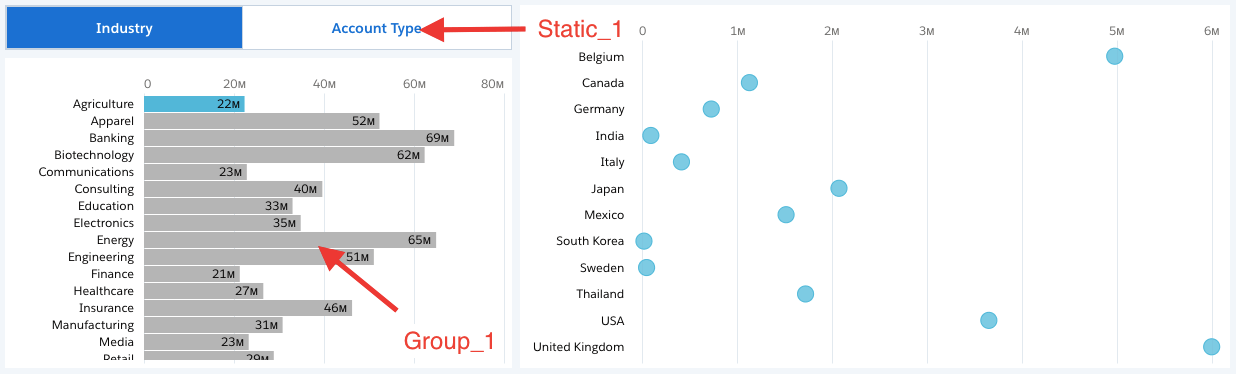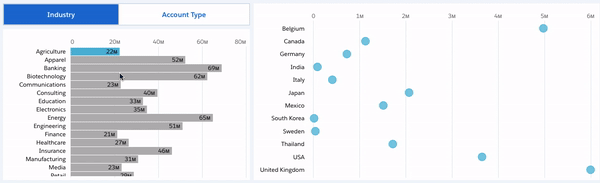Einstein Analytics: Demystifying Bindings – Part 5

28. March 2019 0

It’s time for the fifth part of this Demystifying Bindings blog series and this binding type is one of the advanced possibilities in Einstein Analytics, I am talking about nested bindings. I am sure they are many variations of use cases where nested bindings are needed but in this blog, I will focus on using nested bindings in a SAQL filter.

What is a nested binding?

First of all, it’s probably appropriate to explain what a nested binding is. Well, we have already covered what a binding is in this blog series; a way to make your step or widget dynamic based on parameters from a source step. A nested binding is a binding within a binding. To be honest nested bindings are in my experience not commonly used but you do occasionally find those edge cases where a nested binding are just what is needed.

Prerequisites for the example

The use case is to have a bar chart where the grouping is bound to a static step. This we covered in part 1 of this blog series or you can also look at the blog I wrote on static steps.

The static step called Static_1 looks like this:

```"values": [
{
"display": "Industry",
"value": "Industry"
},
{
"display": "Account Type",
"value": "Account_Type"
}
]```

And the step Group_1 looks like this with the binding to the above static step – I’ve kept it in compact form:

```{
"measures": [
[
"sum",
"Amount"
]
],
"groups": "{{column(Static_1.selection, [\"value\"]).asObject()}}"
}```

And finally, we have the SAQL step we will be adding a nested binding to, which in my use case is the filter:

```q = load "DTC_Opportunity_SAMPLE";
q = filter q by 'Industry' == "Agriculture";
q = group q by 'Billing_Country';
q = foreach q generate 'Billing_Country' as 'Billing_Country', sum('Amount') as 'sum_Amount';
q = order q by 'Billing_Country' asc;
q = limit q 2000;```

Visually this is how it looks in my example:Anatomy of the nested binding

The idea behind this binding is we want the SAQL step to have a filter that is powered by our aggregateflex step (compact form), however, this step is already dynamic, so we need to make sure that the binding in the SAQL step takes this into account.

If we think back to the second part of this blog series, a filter binding looks like this:

`q = filter q by {{cell(Step_1.selection, 0, \"column name\").asEquality(\"Field API Name\")}};`

It’s important to remember that ‘Step_1’ is the source step, ‘column name’ is the name of the column from where we want to grab the value. Since we have defined ‘0’ as the row and it’s a cell selection then we will be looking at the very first value. Finally, in the .asEquality() we have the field or column in the dataset that we want to match the value from the ‘column name’ with.

Below you will see the step with the nested filter binding:

```q = load "DTC_Opportunity_SAMPLE";
q = filter q by {{column(Group_1.selection, column(Static_1.selection, [\"value\"])).asEquality(cell(Static_1.selection, 0, \"value\"))}};
q = group q by 'Billing_Country';
q = foreach q generate 'Billing_Country' as 'Billing_Country', sum('Amount') as 'sum_Amount';
q = order q by 'Billing_Country' asc;
q = limit q 2000;```

Let’s break the nested binding down. First, if we look at below we are binding the filter to the selection from our chart; Group_1 is our source step. Now we do need to define the column we want to grab from the source step and what it should be equal to, which I for now just have filled with static parameters – the industry.

`q = filter q by {{column(Group_1.selection, [\"Industry\"])).asEquality(\"Industry\")}};`

Now we know that we cannot use the static parameters, and looking back to our step ‘Group_1’ this step is already dynamic, hence we need to replace the static parameters with dynamic values.

If we look at the ‘column name’ in the data selection this is powered by the group selection binding we have in the step ‘Group_1’ hence we need to add in the same data selection in our filter binding. Just remember we do not need to add the data serialization in this nested binding, we just want to take the data selection. We now end up with the following:

`q = filter q by {{column(Group_1.selection, column(Static_1.selection, [\"value\"])).asEquality(\"Industry\")}};`

The final thing we have to do is to define the column name we want to apply the filter selection to. As you saw with the static example we were matching to the ‘Industry’ column. If I in my ‘Static_1’ step select ‘Industry’ for my ‘Group_1’ step then my nested binding will work. However, if I select ‘Account Type’ then the value we are parsing is not gonna find a match, hence we need to make this part dynamic as well and add another nested binding looking at the same static step as we did with the other nested binding.

`q = filter q by {{column(Group_1.selection, column(Static_1.selection, [\"value\"])).asEquality(cell(Static_1.selection, 0, \"value\"))}};`

And that should be it. Now you can select ‘Industry’ or ‘Account Type’ from your toggle, which changes the grouping in the bar chart and then apply the selected bar as a filter in the dot plot chart.If you want to use bindings in compare tables, that is what we will be looking at next.

This site uses Akismet to reduce spam. Learn how your comment data is processed.